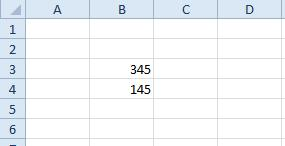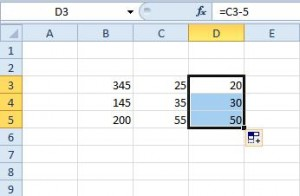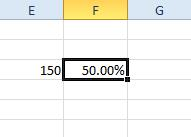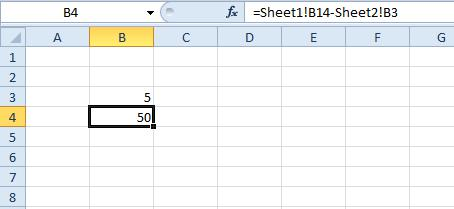# How to Subtract in Excel with a Formula

Excel is a spreadsheet application that has a plethora of functions. Yet, the software doesn’t have a subtraction function, which seems an obvious one to include. As such, Excel users manually enter formulas in the function bar to subtract numbers.Even though this might seem like a major inconvenience, it’s quite simple to do without a subtraction function. In this article, we’ll cover some of the various ways you can deduct values in Excel spreadsheets.

## Subtracting in Excel using Formulas

Given the multitude of ways you might need to subtract values and cells in Excel,

### Subtract by including Values in the Formula

You don’t need to enter any values in spreadsheet cells to subtract numbers. Instead, you can include the values to subtract within the formula itself.

1. First, select a cell to add the formula to.
2. Then click in the Excel function bar and input ‘=‘ followed by the values you need to deduct. For example, input ‘=25-5‘ in the function bar and press Enter. The formula’s cell will return the value 20.

However, most Excel users will need to subtract numbers entered in spreadsheet columns and rows. To subtract cell values, you’ll need to include their row and column references in the formula instead.

1. For example, enter the values ‘345‘ and ‘145‘ in cells B3 and B4 in a blank Excel spreadsheet as in the snapshot below.2. Now, select cell B5 and click in the fx bar to enter a formula. Input the formula ‘=B3-B4‘ and press the Return key. B5 will now return the value 200 as shown below.### Subtract One Number from Each Value within a Cell Range

If you need to subtract a single value from each number within a cell range, you can copy the formula to other cells.

1. For example, enter the values ‘25,’ ‘35‘ and ‘55‘ in cells C3:C5.
2. Then, select cell D3, enter the formula ‘=C3-5‘ in the function bar and press the Return key. D3 will now subtract 5 from 25 in cell C3. Copy the formula in D3 to the other cells below it by selecting D3, clicking the bottom right corner of the cell and dragging it over the ones below as shown below.
3. Now, the cells directly below D3 also subtract 5 from the numbers in C4 and C5.### Subtract a Cell Range Total from a Single Value

What if you need to subtract a column total for a group of cells from a single value? One way to do that would be to enter a SUM function in a cell that adds the range together, and then subtract that total with a separate formula. However, you can also subtract a cell range total by including SUM within a formula.

1. For example, enter the values ‘45,’ ‘55‘ and ‘75‘ in the cells B7 to B9.
2. Then, input ‘200‘ in cell B11. Select cell B12, click in the fx bar and input ‘=B11-SUM(B7:B9)‘ in the formula bar. Cell B12 will then return the value of 25 as shown directly below. That effectively deducts the total of cells B7:B9 from 200 in cell B11.### Subtract Two or More Total Cell Range Values

You can also subtract cell range totals without entering SUM functions in the spreadsheet first. Instead, add the cell range references to a formula and subtract them.

1. For example, enter the numbers ‘25,’ ‘15‘ and ‘35‘ in cells C7:C9. Now you can deduct the total of the C7:C9 cell range from the B7:B9 range entered earlier.
2. Select C11 as the cell to add the formula to, and then input ‘=SUM(B7:B9)-SUM(C7:C9)‘ in the function bar. Press Enter to add the function to the spreadsheet, which will return the value 100 in C11. So, that’s deducting the C7: C9 cell range from the B7:B9 cell range total, which is otherwise 175 – 75.### Subtracting Percentage Values from Figures

To subtract a percentage value, such as 50%, from a number, you’ll need to enter the value in a cell with percentage format. Then you can add a formula that subtracts the percentage from a number in another cell.

1. As an example, enter the value ‘150‘ in cell E3. You should convert cell F3 to percentage format by right-clicking the cell and selecting Format Cells > Number > Percentage > OK. Enter ‘50‘ in F3, which will then be in percentage format as shown directly below.2. Now you can add a formula that subtracts 50% from 150, Click cell G3 and enter the formula ‘=E3-(F3*E3)‘ in the function bar. Cell G3 will return a value of 75, which is 50% of 150. Thus, the formula has deducted 75 from 150.### Subtracting Values Across Multiple Worksheets

Excel doesn’t limit you to subtracting values within single worksheets. As such, you can subtract cell numbers in one worksheet from those in another.

1. For an example, enter ’55’ in cell B14 in Sheet1 of a spreadsheet.
2. Then, click the Sheet2 tab at the bottom of the application’s window to open a blank spreadsheet. Enter ‘5’ in cell B3 of Sheet2. So this formula will subtract B3 in Sheet2 from B14 in Sheet1.
3. Now, click B4 in Sheet2 to add the formula to that cell. Enter the formula ‘=Sheet1!B14-Sheet2!B3‘ in Excel’s function bar and press Return. Cell B4 will now deduct 5 in Sheet2’s B3 from B14’s value in Sheet1. The cell returns a value of, as you probably guessed, 50.## Subtracting in Excel

So, those are some of the subtraction formulas that subtract values in Excel spreadsheets. You can copy the formulas above to your spreadsheets with the Ctrl + C and Ctrl + V hotkeys and edit their cell references as required. Check out this Tech Junkie guide if you need to subtract dates in Excel.

Do you know of other ways to subtract values in Excel? Share your experience below.

Disclaimer: Some pages on this site may include an affiliate link. This does not effect our editorial in any way.

Todays Highlights